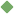geometric_mean 1. [ noun ] (mathematics,statistics) the mean of n numbers expressed as the n-th root of their product Related terms: mean statistics To share this definition press "text" (Facebook, Twitter) or "link" (blog, mail) then paste text link Similar spelling: geometrician   geometric_pace   Geometridae   geometrical   geometric_series   geometrically   geometrid_moth